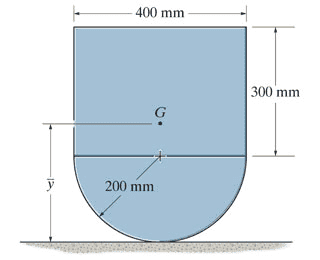# Determine the mass moment of inertia of the assembly

• Pascal1p
Use the correct value for ##I_{cm}## and your answer will be correct.In summary, the conversation discusses determining the location of the center of mass and calculating the moment of inertia for an assembly consisting of a block and a semicylinder. The parallel axis theorem and moments of inertia for a plate and semicylinder are mentioned. The attempt at a solution involves finding the center of mass and using the parallel axis theorem to calculate the moment of inertia. However, the calculation for the moment of inertia of the semicylinder is incorrect as it uses the wrong value for the rotational inertia about the center of mass. The correct value for the moment of inertia of the semicylinder should be used to obtain the

## Homework StatementDetermine the location of y of the center of mass G of the assembly and then calculate the moment of inertia about an axis perpendicular to the page and passing through G. The block has a mass of 3 kg and the mass of the semicylinder is 5 kg.

## Homework Equations

-parallel axis theorem
-moment of inertia plate
-moment of inertia semicylinder
- y= (Σydm)/(Σdm)

## The Attempt at a Solution

The block has center at y=350, dm= 3, ydm= 1050
The semicylinder has center at y=115,117, dm=5 and ydm= 575,585
So y(center)= (1050+575,585)/(8)= 203,198 mm

(answer in back of book is 203 mm, so this seems correct)

now I(block) = (1/12)*m*(a^2+b^2)+ md^2= (1/12)*3*(0.3^2+0.4^2) + 3*(0.203198-0.350)^2
=> 0.1271524816 kg*m^2 (as you can see I converted mm in m, which explains the 0.203198 instead of 203.198 for example)
now I(semicylinder)= (1/2)*m*R^2 + md^2= (1/2)*5*(0.2)^2+ 5* (0.203198-0.115117)^2
=> 0.13879 kg*m^2 (I heard, but also verified myself that the moment of inertia for semicylinder is same as for full cylinder, hence 1/2*m*R^2.

The answer should be 0.230 kg* m^2
but i get 0.266 kg* m^2

So what did I do wrong? Is the moment of inertia for semicylinder not correct afterall?

EDIT: When getting ##I## for the semi-cylinder, does ##(1/2)MR^2## give you the rotational inertia about the CM of the semi-cylinder?

What does the parallel axis theorem say about which moments of inertia ?

TSny said:
EDIT: When getting ##I## for the semi-cylinder, does ##(1/2)MR^2## give you the rotational inertia about the CM of the semi-cylinder?

Ow, so the moment of inertia for the semi cylinder with (1/2)MR^2 is not around the the center of the semi cylinder but around the center of the full cylinder it would make? So in this case it would be around the z-axis which passes through y=200 mm?

So 0.5*5*(0.2)^2+(0.203-0.2)^2*5= I(cylinder)

Together with the I(plate) gives 0.227. So why is it still off?

BvU said:
What does the parallel axis theorem say about which moments of inertia ?
Meaning you want to make a different kind of calculation. Answer to #4 is yes.

Pascal1p said:
So 0.5*5*(0.2)^2+(0.203-0.2)^2*5= I(cylinder)

Together with the I(plate) gives 0.227. So why is it still off?
The parallel axis theorem ##I = I_{cm} + md^2## involves the rotational inertia about the center of mass of the object, ##I_{cm}##. Your calculation uses ##(1/2)MR^2## instead of ##I_{cm}##.